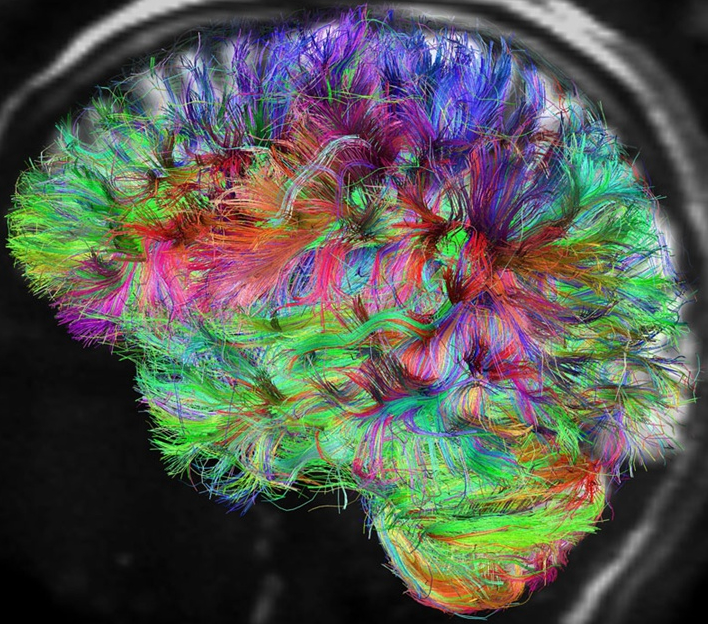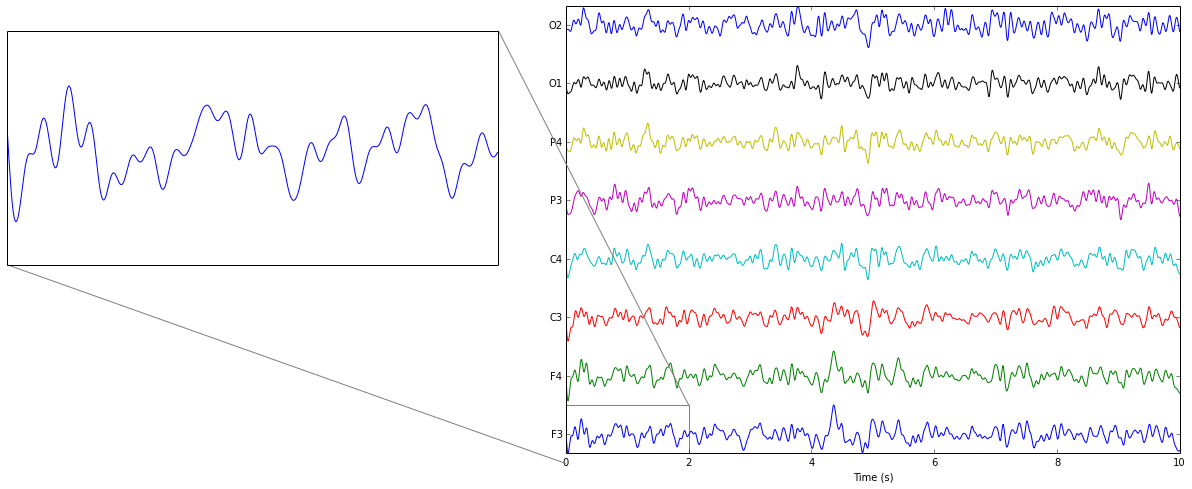# Introduction to Recurrent Networks and Applications in EEG Analysis

Electroencephalography (EEG) records electrical signals from the surface of the scalp that, ideally, provide information about activity in the brain.

Extracting useful information from EEG signals can, however, be extremely challenging.

• EEG signals are measured at the $\mu V$ level, making them very susceptable to noise and artifacts.
• The signals become "blurred" as they travel through the scull, meninges and scalp.
• Only electrical activity near the surface of the scalp and traveling at the correct orientation can be measured.
• This means that EEG really only measures the synchronized firing of populations of neurons.The signals that we are able to get originate from the brain, which is a very sophisticated organ. On the neural scale:

• The human has hundereds of billions of neurons and hundereds of trillions of synaptic connections.
• The interactions between neurons are complex and often involve non-linear electro-chemical processes.Above image borrowed from Discover Magazine: http://discovermagazine.com/2013/jan-feb/36-new-project-maps-the-wiring-of-the-mind

On the cognative scale:

• The brain is continually processing large amounts of information in parallel.
• It is also continually collating information and learning about the past, present and future.Image borrowed from Wikimedia Commons: http://commons.wikimedia.org/wiki/File:Auguste_Rodin-The_Thinker-Legion_of_Honor-Lincoln_Park-San_Francisco.jpg

### So what does this mean for us as data scientists and neural engineers?

Methods for modeling and classifying EEG signals should be

• robust to noise
• able to model spatial patterns (across electrode sites)
• able to model temporal patterns (over the course of time)

I posit that Artificial Neural Networks (ANN) and, specifically, Recurrent Artificial Neural Networks (RNN), are well-suited for this role.

In this talk we will begin to explore methods for capturing spatiotemporal information using neural nets and give some examples of how this can be used to analize EEG signals.

Although we are focusing on EEG, these methods should be generally useful for analyzing other time-series and time-varying signals

Let's begin with some prerequisites.

In :
# plotting
%matplotlib inline
import matplotlib.pyplot as plt
from mpl_toolkits.axes_grid1.inset_locator import zoomed_inset_axes
from mpl_toolkits.axes_grid1.inset_locator import mark_inset

# animation
from JSAnimation.IPython_display import display_animation
from matplotlib import animation

# matrices
import numpy as np
import scipy.sparse as sparsem
import scipy.sparse.linalg as sparsela

# JavaScript object notation
import json

# bandpass filtering, should be in tarball
import bandpass as bp

In :
def addBias(m):
"""Add a column of ones for a bias term.
"""
m1 = np.empty((m.shape, m.shape+1))
m1[:,:-1] = m
m1[:,-1] = 1.0
return m1

In :
def loadData(fileName='s20-gammasys-gifford-unimpaired.json'):
"""Load some resting state EEG data.
"""
dataHandle = open(fileName, 'r')
dataHandle.close()

data = data # pull out resting state session
sampRate = data['sample rate']
chanNames = data['channels']
nChan = len(chanNames)
sig = np.array(data['eeg']['trial 1']).T
sig = sig[:,:nChan] # omit trigger channel

return sig, chanNames, sampRate


$\newcommand{\transpose}{\mathsf{T}}$ $\newcommand{\Mat}{\mathbf{#1}}$

Next, let's generate some data to play with. Rows are observations and columns are channels.

sig is the signal we are interested in exploring. In this case, we examine 10 seconds of resting-state EEG. A bandpass filter is applied from 1-12Hz. This gives us a relatively smooth signal. In more realistic applications, we would want a wider passband.

time is simply a column vector $\left[ 1, \ldots, N \right]^\transpose$ denoting each timestep of our signal.

window gives the indices for the first 3 seconds of data. We will use this window to train our models and the remaining data to examine generalization.

In :
if False:
# sinusoid data for testing
time = np.arange(0.0, 20.0*np.pi, 0.1)[:,None]
sig = np.sin(time) #+ np.random.normal(scale=0.15, size=tFull.shape)
window = np.arange(int((4.0*np.pi)/0.1))
else:
# EEG data
filt = bp.IIRFilter(1.0, 12.0, sampRate=sampRate, order=3)
sig = filt.filter(sig)
sig = sig[:(10.0*sampRate),:]
time = np.arange(0.0, 10.0, 1.0/sampRate)[:,None]
winWidth = 2.0
window = np.arange(int(winWidth*sampRate))

# for separating signal channels
scale = np.max(np.abs(sig))
sep = np.arange(sig.shape) * 2.0 * scale

In :
fig = plt.figure(figsize=(11,8))
ax.plot(time, sig+sep[None,:])
ax.set_yticks(sep)
ax.set_yticklabels(chanNames)
ax.set_xlabel('Time (s)')
ax.autoscale(tight=True)

# zoomed inset plot
subx1,subx2 = (0.0,winWidth)
#suby1,suby2 = (-scale,sep[-1]+scale)
suby1,suby2 = (-scale,scale)
axins = zoomed_inset_axes(ax, zoom=4.0,
bbox_to_anchor=(-1.0,sep[-1]),
bbox_transform=ax.transData)
axins.plot(time[window], sig[window]+sep[None,:])

axins.set_xlim(subx1,subx2)
axins.set_ylim(suby1,suby2)
axins.get_xaxis().set_visible(False)
axins.get_yaxis().set_visible(False)
mark_inset(ax,axins, loc1=3, loc2=1, fc="none", ec="0.5");In :
# standardize using training data
time -= np.mean(time[window], axis=0)
time /= np.std(time[window], axis=0)
sig -= np.mean(sig[window], axis=0)
sig /= np.std(sig[window], axis=0)


First, let's look at the style of two-layer neural nets that we have already talked about. An example of such a network is depicted below.Say that the network inputs are concatenated into a matrix $\Mat{X}$ with rows being observations and columns being dimensions. Also, let $\Mat{H}$ and $\Mat{V}$ be the weight matrices for the hidden and visible layers, respectively, and $\phi$ be our choice of sigmoid. The output of the network's hidden layer is

$\Mat{Z} = \phi(\Mat{\bar{X}}\Mat{H})$

and the final network output is

$\Mat{Y} = \Mat{\bar{Z}}\Mat{V}$

where a bar above a matrix denotes that a column of ones has been added for a constant bias term. These equations are also known as the forward pass.

Since these networks are directed and acyclic, they have a topological ordering. This means that they can be arranged so that all connections move in the forward direction from one layer to the next. For this reason, they are referred to as feedforward networks.

Below, we have a simple implementation of a two-layer feedforward network that is trained using stochastic gradient descent.

In :
class FFNN(object):
"""Feed-Forward Neural Network
"""
def __init__(self, x, g, nHidden=10, *args, **kwargs):
self.nIn = x.shape
self.nOut = g.shape
self.nHidden = nHidden

hwRange = np.sqrt(3.0 / (self.nIn+1))
self.hw = np.random.uniform(-hwRange, hwRange,
size=(self.nIn+1, self.nHidden))

vwRange = np.sqrt(3.0 / (self.nHidden+1))
self.vw = np.random.uniform(-vwRange, vwRange,
size=(self.nHidden+1, self.nOut))

self.train(x, g, *args, **kwargs)

def eval(self, x):
z = np.tanh(x1.dot(self.hw))
return z1.dot(self.vw)

def error(self, x, g):
y = self.eval(x)
return np.mean((g-y)**2)

z  = np.tanh(x1.dot(self.hw))
zPrime = 1.0-z**2
y = z1.dot(self.vw)
delta = 2.0 * (y - g) / g.size

hg = x1.T.dot(delta.dot(self.vw[:-1,:].T) * zPrime)
vg = z1.T.dot(delta)

return hg, vg

def train(self, x, g, hLearn=0.15, vLearn=0.1, learnDecay=0.0,
batchSize=30, iterations=2000, verbose=False):
for i in xrange(iterations):
if verbose:
print i, self.error(x, g), np.array((hLearn, vLearn)) * np.exp(-i*learnDecay)
indices = np.random.permutation(np.arange(x.shape))[:batchSize]
self.hw[...] -= hg * hLearn * np.exp(-i*learnDecay)
self.vw[...] -= vg * vLearn * np.exp(-i*learnDecay)


Next, let's use our feedforward network to fit our EEG signal in the same fashion that we have fit curves before. So, the elements of the input matrix are time,

$\Mat{X}_{t,1} = t$

for $t = 1, \ldots, N$, where $N$ is the length of our training signal. The elements of the target matrix are then the signal values,

$\Mat{G}_{t,i} = \Mat{s}_i(t)$,

for each channel indexed by $i = 1, \ldots, 8$ and where $\Mat{s}(t)$ is the row vector of EEG signal voltages at time $t$.

In :
xFull = time
gFull = sig

x = xFull[window]
g = gFull[window]
t = time[window]

net = FFNN(x, g, nHidden=30, hLearn=0.7, vLearn=0.6, learnDecay=3.0e-5,
batchSize=50, iterations=40000, verbose=False)
print net.error(x, g)

yFull = net.eval(xFull)
y = yFull[window]

fig = plt.figure(figsize=(11,8))

gline, = ax.plot(t, g[:,1], linewidth=3, color='blue')
yline, = ax.plot(t, y[:,1], linewidth=2, color='red')
ax.set_ylim(-2.5, 2.5)

def update(win):
x = xFull[win]
g = gFull[win]
t = time[win]
y = yFull[win]

gline.set_ydata(g[:,1])
gline.set_xdata(t)

yline.set_ydata(y[:,1])
yline.set_xdata(t)

ax.set_xlim((t, t[-1]))
return gline, yline, ax

def dataGen(win):
win = np.array(win, copy=True)
def dataGenWrapped(win=win):
#while win[-1]+3 < len(x):
while True:
win += 20
if win[-1] >= len(xFull):
win -= win
yield win
return dataGenWrapped

ani = animation.FuncAnimation(fig, update, dataGen(window), interval=80)
display_animation(ani, default_mode='once')

0.250105932159


Out:

Once Loop Reflect